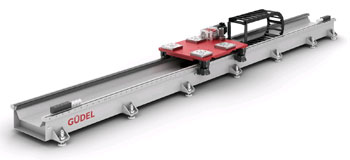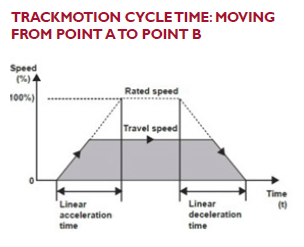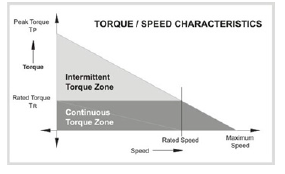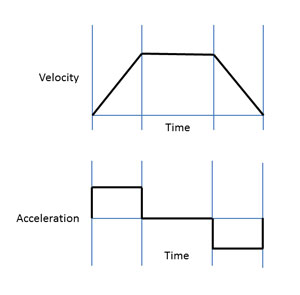• Font Size:
• A
• A
• A

Robotics Tech Papers

Tech Papers# Cycle Times for Trackmotion Robotic Cells

Güdel, Inc.CYCLE TIMES FOR TRACKMOTION ROBOTIC CELLS
Placing a robot on a track greatly expands its reach envelope, potentially allowing it to tend many more machines or to transfer materials across a much greater distance. For example, instead of three robots each tending a machine at separate locations, a single robot on a track could potentially tend all three, saving money and increasing efficiency. Or, a single material handling robot on a rail could replace multiple robots with intermediate handoff locations.

MINIMIZING CYCLE TIME : GENERAL PRINCIPLES
The overall cycle time of a cell is defined as the time it takes to produce a single unit. There are many factors that affect the cycle time of a robotic cell.

1. Cell Layout. The layout of the workcell will have a major effect on the overall cycle time. The placement of the robot and other equipment, such as machine tools, conveyors, and pallet racks, should be done with the sequence of operations in mind.

For a work cell utilizing a TrackMotion Linear Axis, first try to minimize the distance that the robot must move along the track between one step and the next. For a work cell with multiple operations, place each operation as close to the previous operation as possible.

2. Robot Motions. Reducing or removing robot motions that are unnecessary or excessive is the next step. Examine intermediate points in the robot path to determine if movements and rotations can be reduced or eliminated.

Some robot controllers allow for easy optimization of robot motions. For example, changing the path accuracy settings to coarse instead of fine for motions which do not require precision allows for increased speed.

Where allowable, increase the acceleration/deceleration and velocity settings to their maximum levels, except where this might be detrimental to the payload (such as for fragile, long, or large parts). This includes both robot movements and the 7th axis movements. (Ensure that 7th axis acceleration and velocity settings are within design parameters, as will be described below.)

Finally, attempt to perform more than one robot operation in parallel instead of in sequence. For example, opening an end effector during an approach motion instead of waiting until the motion has stopped.

TRACKMOTION CYCLE TIME: MOVING FROM POINT A TO POINT BFor a robot on a track, minimizing the distance between operations is the first step to reduce overall cycle time. Once this has been done, there are a number of factors which will determine how quickly the robot is able to move through its sequence of operations.

• The carriage motion profile1 describes the speed and acceleration of the robot carriage over time.
• To move from point A to point B, the carriage accelerates to a certain velocity, maintains that travel velocity for some amount of time (greater than or equal to zero), and then decelerates to a stop.
• On the speed-time graph this looks like a trapezoid, so this is known as a “trapezoidal” motion profile.
• If the travel distance is sufficiently long, greater than the “critical distance,” the carriage will reach its maximum velocity (or rated speed).

Maximum velocity is a function of several factors, including the rated speed of the servo motor, the rated speed of the gearbox, and the pinion size. For longer tracks, increasing the maximum velocity of the 7th axis can significantly decrease the cycle time of your cell.

Determining Maximum Velocity. For a TrackMotion linear axis driven by a Güdel rack & pinion system, the maximum velocity is determined simply by the effective pitch radius of the pinion gear and the maximum angular velocity at which it is able to turn.

The pinion pitch radii are 25.47mm for the Güdel TMF-1 and TMF-2, 33.96mm for the TMF-3, and 42.44mm for the TMF-4.

Gearbox rating & ratio: Each gearbox will have a maximum allowable input speed. For the Güdel AE060 gearbox (used for TMF-1 and TMF-2) this is 6000 RPM (628.3 rad/s). For the AE090 (TMF-3) and AE120 (TMF-4) gearboxes it is 4500 RPM (471.2 rad/s). Also, each gearbox is available in ratios from 2:1 to 24:1. Dividing the maximum input speed by the gear ratio i will provide the maximum output speed at which the pinion gear will turn.

Therefore, in order to increase the maximum velocity of a given system, choose a smaller gearbox ratio. (However, this will have an inverse effect on maximum acceleration, as described below.)

Based on the information above, the maximum possible velocity (using a gearbox ratio 2:1 and the maximum gearbox input RPM) is 8.0 m/s for the TMF-1, TMF-2, and TMF-3, and 10.0 m/s for TMF-4. However, it is not advisable that the gearbox be operated at maximum input speed. Also, finding a servo motor which can provide the required torque at such high speeds may be problematic, if not impossible.

Therefore it is safe to assume that the actual maximum velocity will be no more than about 60-70% of those theoretical values. Finally, keep in mind that choosing a small gearbox ratio (such as 2:1) will have a negative effect on the maximum possible acceleration, as described below.

Determining Maximum Acceleration. Determining the maximum possible acceleration of the carriage requires quite a bit more information. According to Newton’s famous equation, acceleration equals force divided by mass. The forces acting upon the carriage include both the force between the pinion and the rack as well as various frictional forces: the rolling resistance of the rollers, friction from the guideway scrapers and lubrication system, etc. The mass of the system includes the carriage itself, the robot on the carriage, as well as everything else that is connected to it (cables, connectors, etc.) Also, because the carriage involves rotational elements (rollers, motor shaft, couplings, gearbox elements, pinion), there is also rotational momentum and rotational friction which must be factored into the equations of acceleration.

For the sake of simplicity, let us focus on the three main variables affecting maximum carriage acceleration: robot mass, servo motor torque, and gearbox ratio.

Robot Mass: For most TrackMotion systems, the most significant contribution to mass will be the robot. Therefore, choosing the smallest robot which can perform the required tasks is essential for maximizing the acceleration of the system. This also applies to any robot riser or payload attached to the carriage. The lighter the overall system, the more quickly it can accelerate with a given applied force.

Servo Motor Torque: There are a wide range of servo motors which are compatible with Güdel gearboxes. Each motor will have its own speed-torque characteristics, usually illustrated by a speed/torque graph. For electric motors, maximum torque will decrease as the speed of the output shaft increases.Servo motors typically have a “peak” or intermittent torque capacity, as well as a rated torque capacity, for continuous operation, for a given speed. The motor can operate for an indefinite period within the “continuous” zone without overheating. Therefore the motor’s RMS speed and torque must lie within the continuous region.

To increase the acceleration of the carriage system, choose a servo motor with a high intermittent torque rating. (Keep in mind that the maximum servo motor torque must not exceed the maximum torque rating of the gearbox coupling, or slipping will occur.)

Gearbox Ratio: Because the gearbox reduces the rotational velocity going from the motor to the pinion, it has the effect of increasing the output torque by a corresponding amount.

Therefore, choosing a higher gearbox ratio allows for a higher output torque for a given motor speed. However, as we saw above, increasing the gearbox ratio has the effect of reducing the maximum velocity! There is a trade-off between maximum velocity and maximum acceleration for a given servo motor.

Balancing Speed and Acceleration. What is the ideal tradeoff between speed and acceleration for a particular application? As mentioned above, at travel distances greater than the critical distance, the robot carriage will peak at its maximum velocity Vmax . The critical distance is a function of the maximum velocity and acceleration of the system:

Short Distance Travel: For travel distances less than this critical distance, where the robot carriage never achieves its maximum velocity before decelerating, the travel time is simply a function of distance and the rate of acceleration:

For short distances (below the critical distance), increasing the maximum velocity has no effect on the travel time! Therefore, when travel distances are short enough such that the robot does not reach its maximum speed, the strategy to reduce travel time is to increase acceleration by choosing a servo motor with higher torque, and/or by choosing a higher gearbox ratio. (Keep in mind that maximum acceleration can also be improved by lightening the payload on the carriage, so look for ways to reduce mass wherever possible).

Long Distance Travel: For distances above the critical distance, the travel time is a function of distance, maximum velocity and acceleration.

For travel distances above the critical distance, the first component of this equation will be the larger factor in the travel time. Therefore, once the critical distance has been exceeded, the first strategy to reduce cycle time is to increase maximum velocity by choosing a servo motor with a greater maximum rated speed, and/or by choosing a lower gearbox ratio. Once an attempt has been made to increase the maximum velocity, further cycle time improvements may be achieved by increasing acceleration (as described above).

WORKFLOW SCHEDULING
For robotic cells in which there is more than one operation being performed on each part, there are several decisions which can be made affecting cycle time. First, there is a decision as to whether a forward or a reverse cycle will be implemented. Secondly, implementing a dual gripper instead of a single gripper can greatly reduce cycle time. Lastly, the number of parallel machines simultaneously performing each operation must be determined for optimum efficiency.

Forward or Reverse Cycle. In a “forward cycle”, the cycle begins with all machines empty. The robot picks up a part from the input buffer, carries it to the first machine, waits for the first machine to finish, then carries the part to the next machine (and so on until all processes are completed and the part is dropped off at the output buffer).

In a “reverse cycle”, the cycle begins with all machines except the first one full (the first machine must be empty in order to receive an incoming part). The robot picks up a raw part from the input buffer and carries it to the first machine. Then the robot travels to the last machine, picks up a finished part, and transfers it to the output buffer. Then the robot goes to the second-to-the-last machine, picks up a finished part, and transfers it to the last machine. This process continues until the robot returns to the beginning.

The reverse cycle allows for more machines to be occupied by parts simultaneously, which increases efficiency. However, it increases the overall move distance required by the robot per part.

So, for single-gripper robots, where the processing time for each machine is always less than the time required for the robot to travel from that machine to the next, the forward cycle is preferable. In all other situations, the reverse cycle is more efficient. 2

Dual-Gripper EOAT. Implementing a dual-gripper EOAT can greatly reduce the overall cycle time for most systems in which machine processing time is greater than the robot travel time. A dual-gripper EOAT allows for all machines to be occupied at the beginning of each cycle, and it reduces the overall travel distance per part. The cycle begins with all machines occupied and both grippers empty. The robot picks up a part from the input device with the first gripper and then travels to the first machine.

The EOAT rotates and picks up the completed part at machine #1 with the empty gripper, the EOAT rotates and drops off the new part from the first gripper. Then the robot travels to machine #2, the EOAT rotates and picks up the finished part with the empty gripper, the EOAT rotates and then drops off the part from the first machine. This sequence continues until the robot drops off a finished part to the output device. It then returns to the beginning and starts the sequence over.

Parallel Machines. In situations where one operation has a longer duration than another, the overall efficiency of the cell can be increased by running more than one of the slower operation in parallel, but having multiple machines. For example, if operation #1 takes 10 seconds per part, but operation #2 takes 30 seconds per part, there will always be wasted time as the robot waits for operation #2 to complete, limiting the overall capacity of the system. However, this wasted time can be reduced by adding additional machines performing the slower operation.

CONCLUSION
There are a number of factors affecting the overall cycle time of a robotic cell, from the layout to the selection of components. Making the work cell as compact as possible, so that robot and track movements are minimized, is the first step. Choosing the lightest robot available which can perform the necessary tasks will allow for more rapid carriage acceleration. A Güdel application engineer can assist in the selection of a servo motor and gearbox ratio combination which will provide the correct tradeoff of velocity and acceleration. Finally, where parts are being moved through multiple operations, a dual-gripper EOAT should be considered.

APPENDIX A: TRAPEZOIDAL AND S-CURVE MOTION PROFILES
Trapezoidal Motion Profile. The trapezoidal motion profile is based on the assumption of constant acceleration and deceleration.Looking at a graph of both velocity and acceleration versus time, you can see that there are three distinct travel phases: a first phase of constant acceleration, a second phase of constant velocity (zero acceleration), and a third phase of constant deceleration. Looking at the acceleration graph, you can see that the transition between these phases is instantaneous—the change in acceleration occurs instantly between each phase. The “jerk” is the rate change of acceleration. For trapezoidal motion, the jerk is infinite.

There are drawbacks to designing a system with extremely high jerk, namely the introduction of vibrations or oscillations. For a given load, the higher the jerk (or change in acceleration), the more powerful the vibrations will be, and the larger the number of vibrational modes that will be excited. This may result in increased settling time or reduced accuracy if the vibration frequency matches resonances in the mechanical system. Vibrations will also lead to greater stresses on the system and reduced life due to material fatigue. Therefore another motion profile, known as the S-curve Profile, is often preferred.

S-Curve Profile. The S-curve consists of 7 distinct phases of motion (as opposed the 3 phases of the trapezoidal profile). Phase 1 starts the load from rest at a linearly increasing acceleration until it reaches the maximum acceleration. In phase 2, the profile accelerates at this maximum acceleration rate until it must start decreasing as it approaches maximum velocity. In phase 3 the acceleration linearly decreases until it reaches zero. In phase 4 the velocity is constant, at which point the profile decelerates in a matter symmetric to phases 1-3.

Since trapezoidal profiles spend all their time at full acceleration or full deceleration, they are faster than the S-curve profile. But if the “all on / all off” approach causes an increase in settling time, the advantage is lost. Many times, even a small amount of smoothing between full acceleration and zero acceleration can substantially reduce induced vibration. The specific choice of the form of the S-curve will depend on the mechanical nature of the system and the desired performance specifications.

Credit for the written content of this document is Don Bromley, Applications Engineer, Güdel, Inc.

1 See Appendix A

2 Dawande, M., Geismar, H. N., Sethi, S. P., & Sriskandarajah, C. S. (2005). Sequencing and Scheduling in Robotic Cells: Recent Developments. Journal of Scheduling, 8, 387-426.

4881 Runway Boulevard
Ann Arbor, Michigan 48108 USA
Tel: 734.214.0000